Constructing Quadrilateral - 2 Diagonals and 3 sides given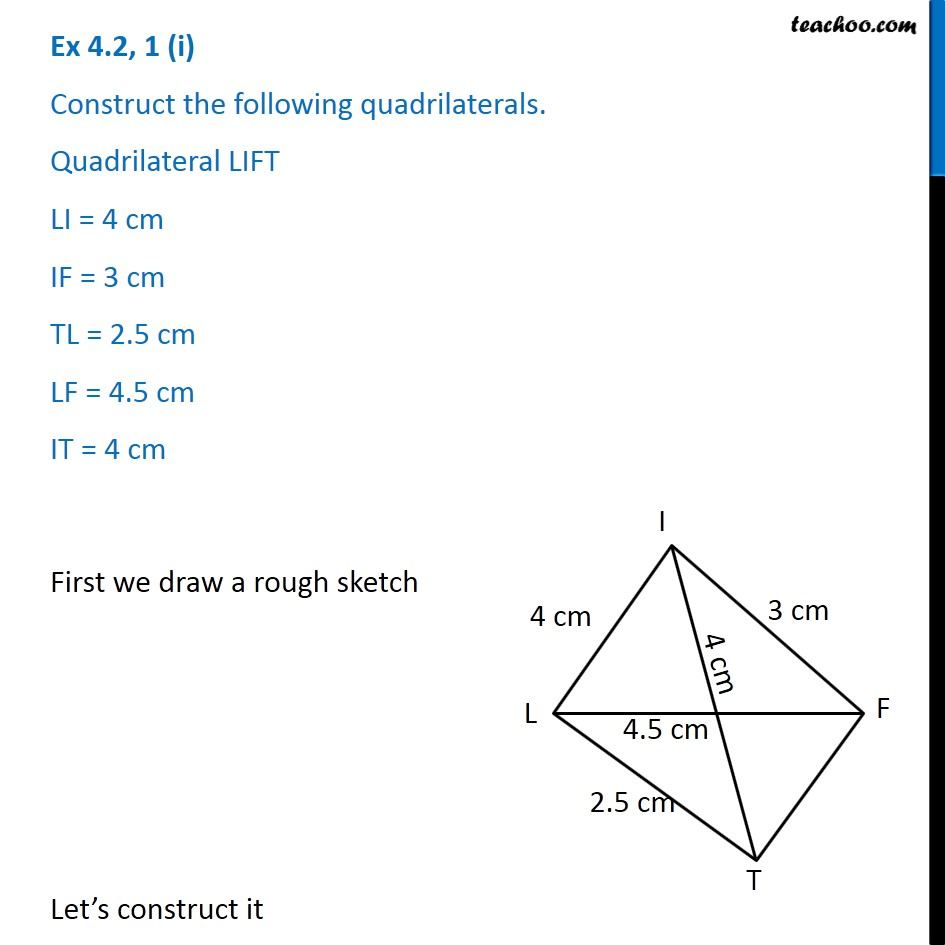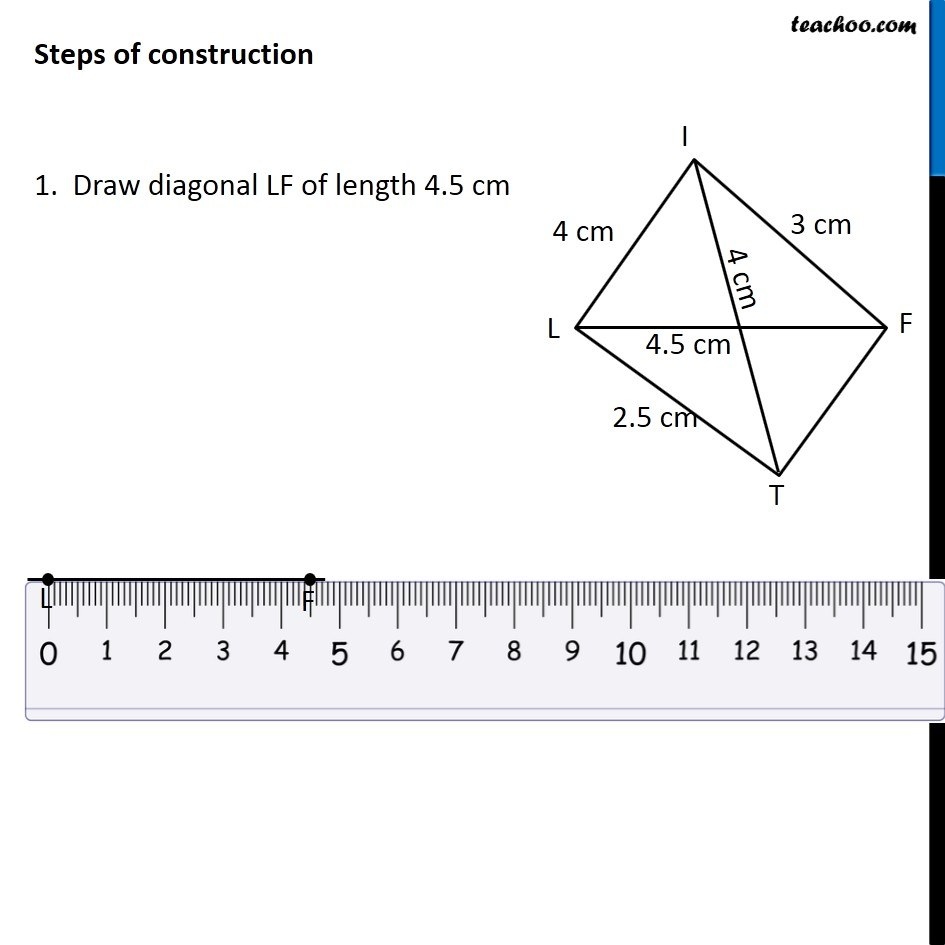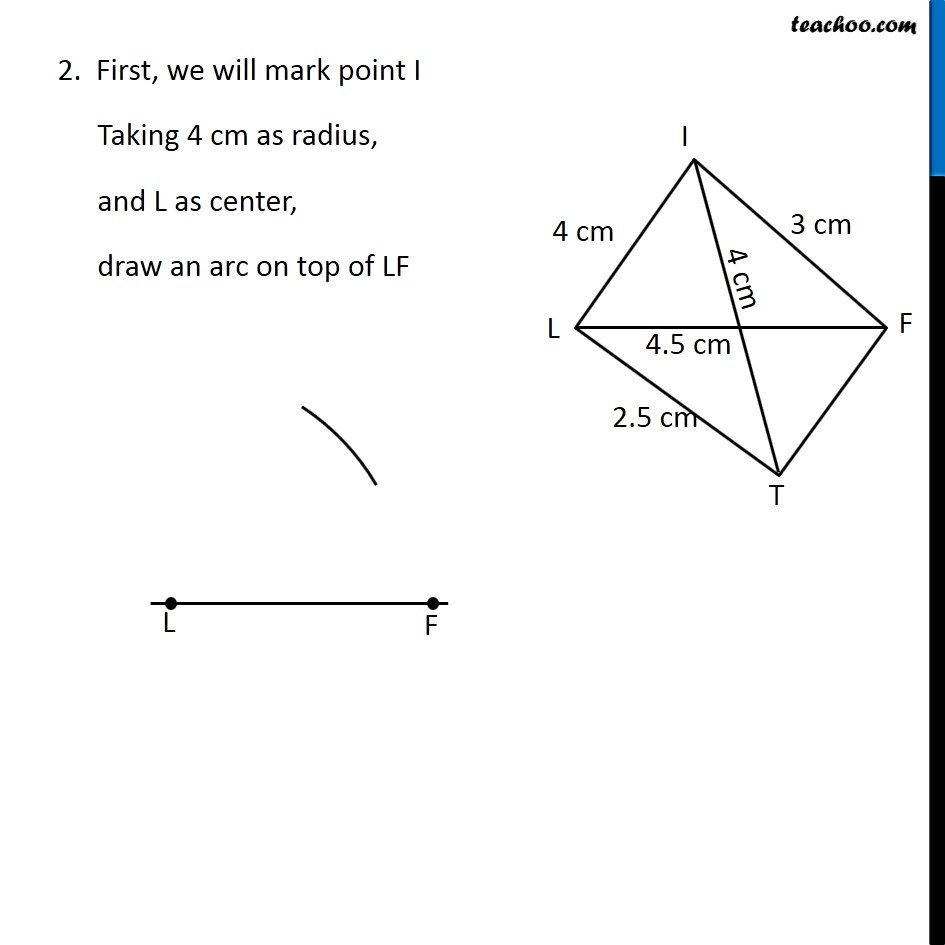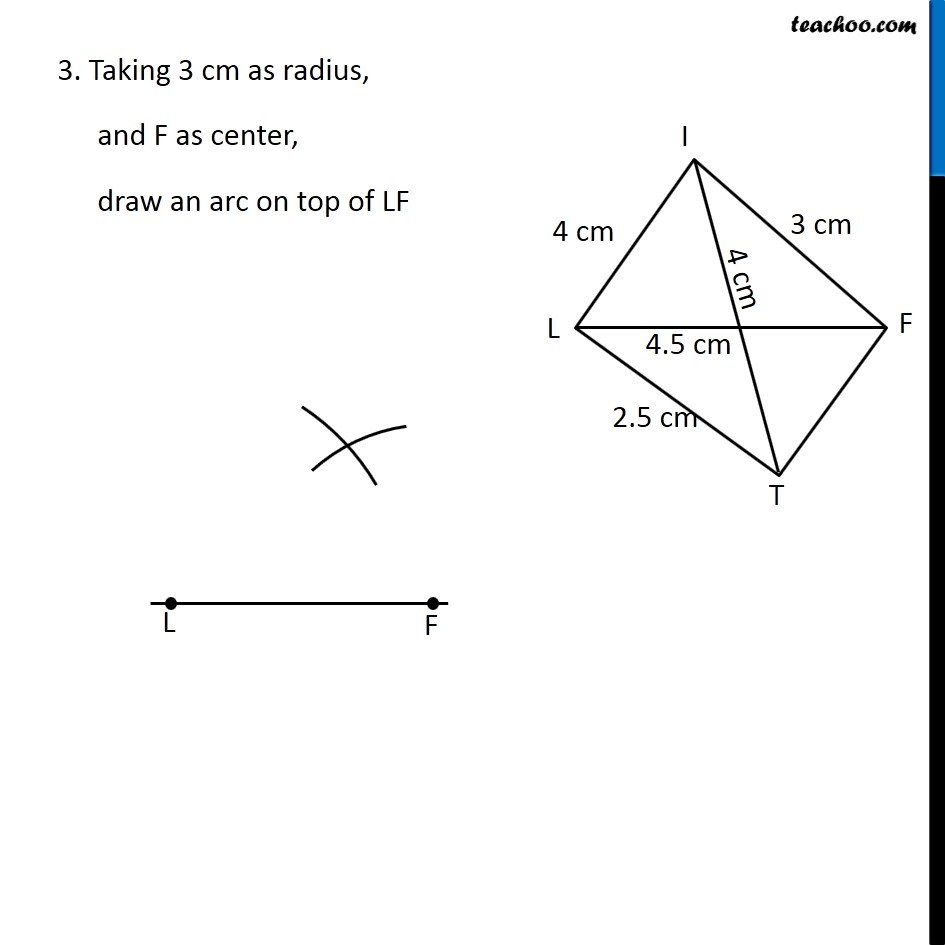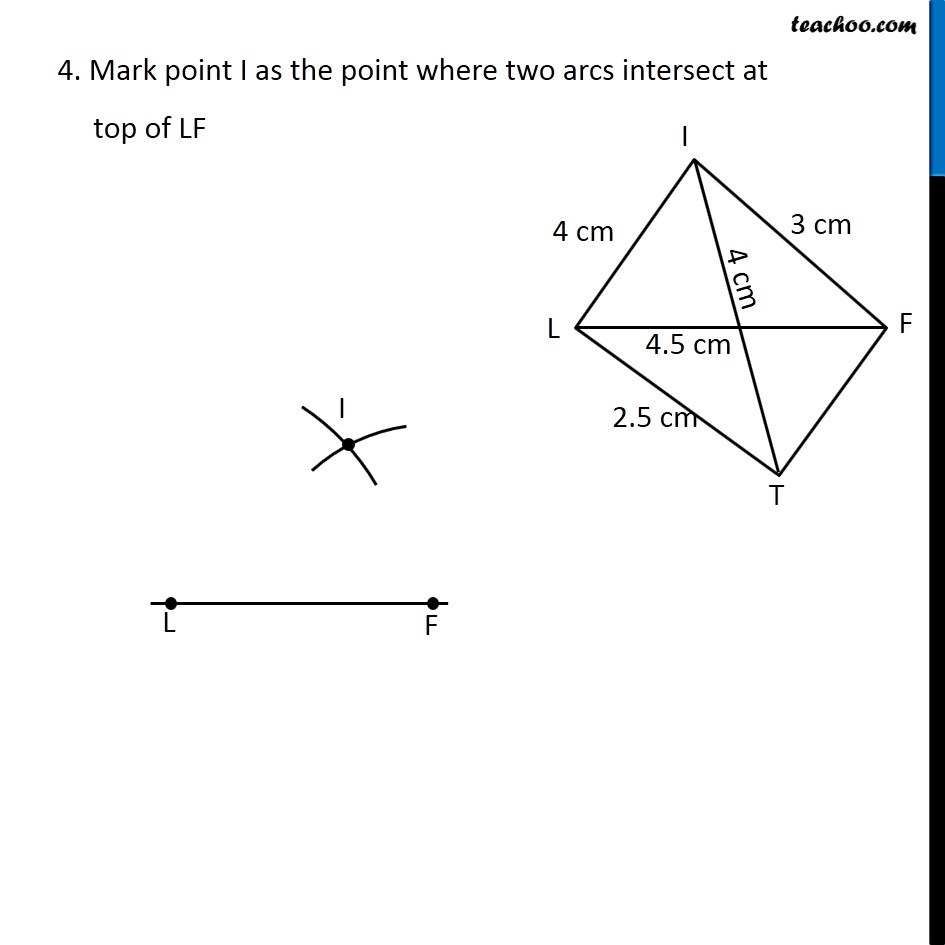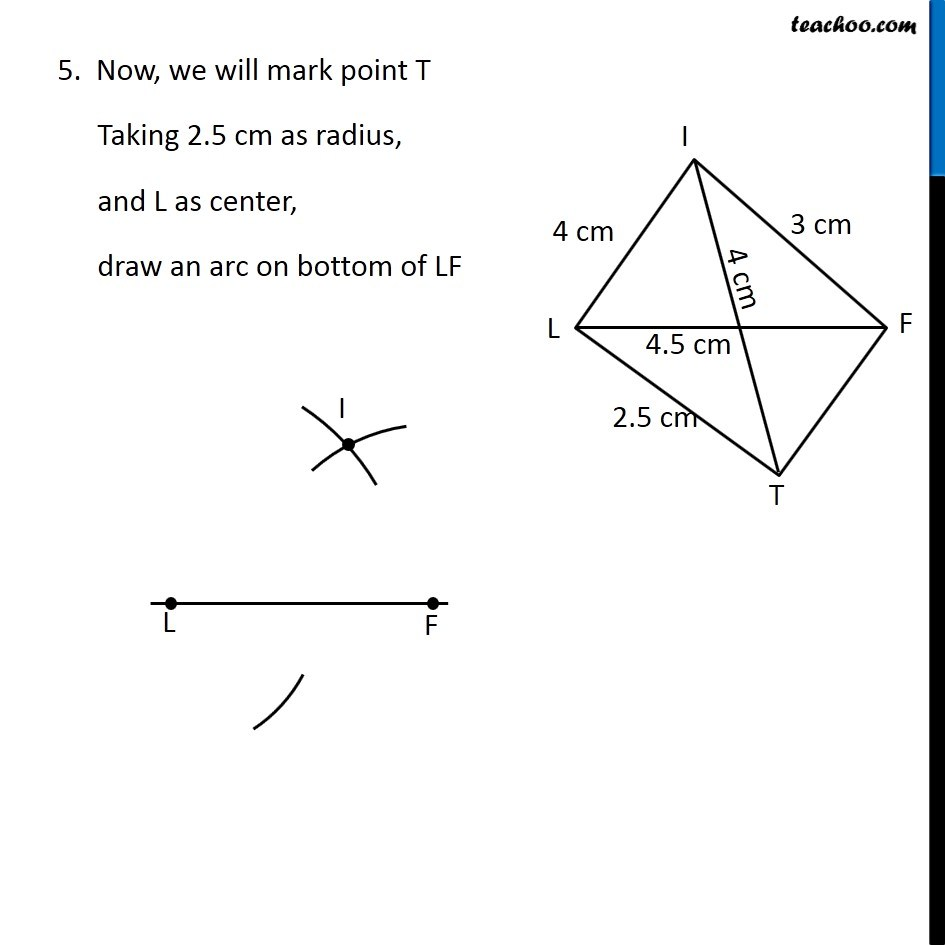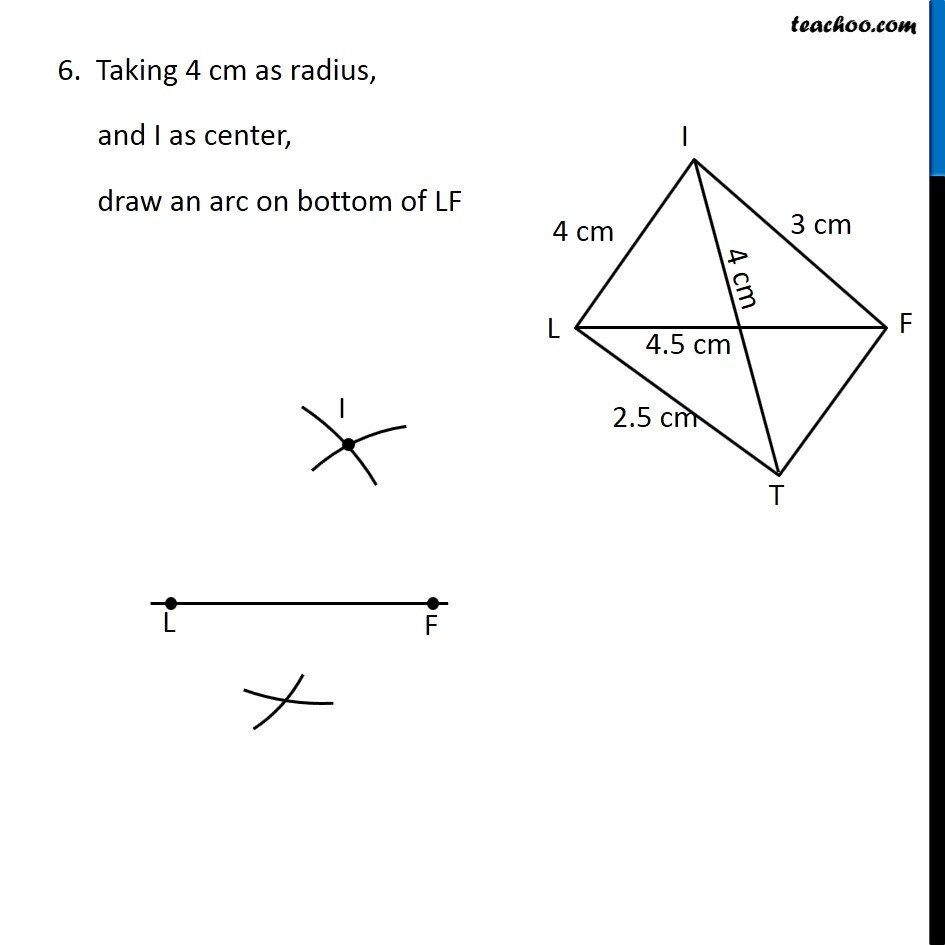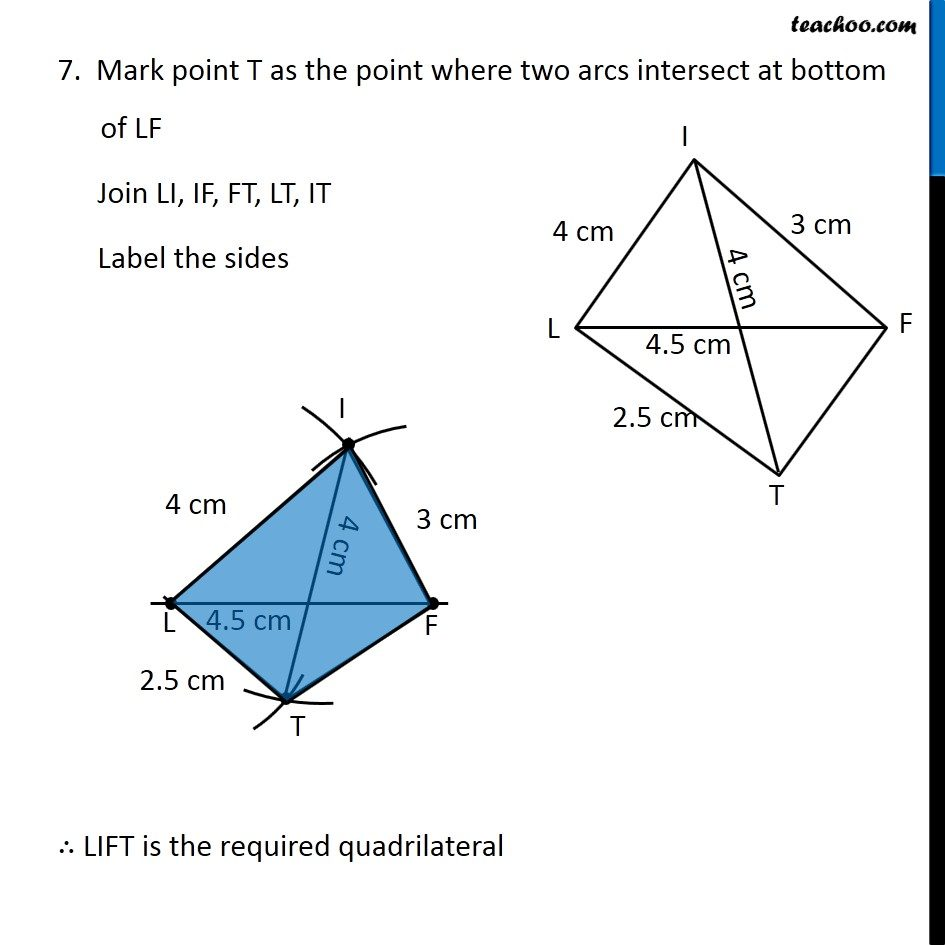Learn in your speed, with individual attention - Teachoo Maths 1-on-1 Class

### Transcript

Question 1 (i) Construct the following quadrilaterals. Quadrilateral LIFT LI = 4 cm IF = 3 cm TL = 2.5 cm LF = 4.5 cm IT = 4 cm First we draw a rough sketch Let’s construct it Steps of construction 1. Draw diagonal LF of length 4.5 cm Steps of construction 1. Draw diagonal LF of length 4.5 cm 3. Taking 3 cm as radius, and F as center, draw an arc on top of LF 4. Mark point I as the point where two arcs intersect at top of LF 5. Now, we will mark point T Taking 2.5 cm as radius, and L as center, draw an arc on bottom of LF 6. Taking 4 cm as radius, and I as center, draw an arc on bottom of LF 7. Mark point T as the point where two arcs intersect at bottom of LF Join LI, IF, FT, LT, IT Label the sides ∴ LIFT is the required quadrilateral